Bayesian Networks in Educational Assessment

Tutorial

Session III: Bayes Net with R

Duanli Yan, Diego Zapata, ETS

Russell Almond, FSU

2021 NCME Tutorial: Bayesian Networks in Educational Assessment

SESSION __ __ __ __ TOPIC __ __ __ __ __ __ __ __ __ __ __ __ __ __ PRESENTERS

Session 1 : Evidence Centered Design Diego Zapata Bayesian Networks

Session 2 : Bayes Net Applications Duanli Yan & ACED: ECD in Action Russell Almond

Session 3 : Bayes Nets with R Russell Almond & Duanli Yan

Session 4 : Refining Bayes Nets with Duanli Yan & Data Russell Almond

# Conditional Probability Tables

• Focus on a child variable
• Child has zero or more parent variables in graph
• For each configuration of parent variables, need conditional probability of each child variable.
• Unconditional probability in the case of no parents
• If there are N parents, each with M states and the child variable has K states, then the number of unconstrained entries in the table is
• M N $$K\-1$$

# Problems

Too many parameters to comfortably elicit

Certain cases might be rare in population $$</span> <span style="color:#000000">Very High </span> <span style="color:#000000">on </span> <span style="color:#000000"> _Skill 1_ </span> <span style="color:#000000"> and </span> <span style="color:#000000">Very Low </span> <span style="color:#000000">on </span> <span style="color:#000000"> _Skill 2_ </span> <span style="color:#000000">$$

Want to capture intuition of experts on how skills interact to generate performace.

# Reduced Parameter Models

• Noisy-and and Noisy-Or models
• NIDA, DINA and Fusion model $$Junker & Sijtsma$$
• Assume binary responses
• Discrete IRT models
• DiBello—Samejima models
• Based on “effective theta” and graded response model
• Compensatory, Conjunctive, Disjunctive and Inhibitor relationships
• CPTtools framework
• Effective theta mapping
• Selectable combination rule
• Selectable link function $$graded response\, normal\, generalized partial credit$$
• For all of these model types, number of parameters grows linearly with number of parents

# Noisy-And (Or)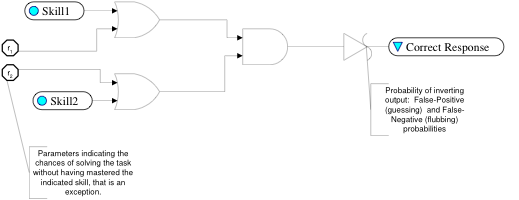All input skills needed to solve problem

Bypass parameter for Skill j , q j

Slip probability $$overall$$, q 0

Probability of correct outcome

NIDA/DINA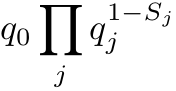# Noisy Min (Max)

• If skills have more than two levels
• Use a cut point to make skill binary $$e\.g\.\, reading skill must be greater than X$$
• Use a Noisy-min model
• Probability of success is determined by the weakest skill
• Noisy-And/Min common in ed. measurement, Noisy-Or/Max common in diagnosis
• Number of parameters is linear in number of parents/states
• Variants of propagation algorithm take advantage of extra Noisy-Or/And independence conditions

# Discrete IRT (2PL) model

• Imagine a case with a single parent and a binary $$correct/incorrect$$ child.
• Map states of parent variable onto a continuous scale: effective theta,
• Plug into IRT equation to get conditional probability of “correct”
• a j _ – _ discrimination parameter
• b j _ – _ difficulty parameter
• 1.7 – Scaling constant $$makes logistic curve look like normal ogive$$

# DiBello–Samejima Models

Single parent version

Map each level of parent state to “effective theta” on IRT $$N\(0\,1$$) scale,

Now plug into Samejima graded response model to get probability of outcome

Uses standard IRT parameters, “difficulty” and “discrimination”

DiBello--Normal model uses regression model rather than graded response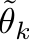# Various Combination Rules

• For Multiple Parents, assign each parent j an effective theta at each level k , .
• Combine Using a Combination Rule $$Structure Function$$
• Possible Structure Functions:
• Compensatory = average
• Conjunctive = min
• Disjunctive = max
• Inhibitor; e.g. level k * on :
• where is some low value.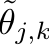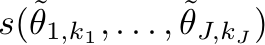# Effective Thetas for Compensatory Relationshipequally spaced normal quantiles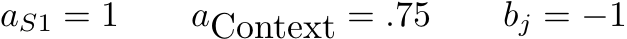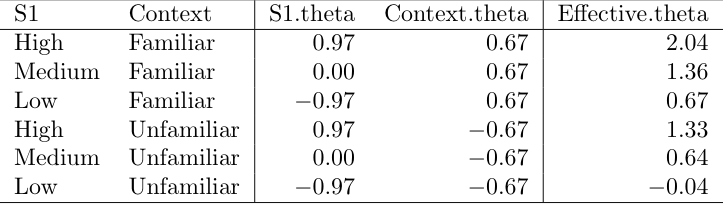# Effective Theta to CPT

Introduce new parameter d inc _ _ as spread between difficulties in Samejima model

b i,Full _ = b_ j _ + d_ inc /2 b j,Partial _ = b_ j _ - d_ inc /2

Conditional probability table for _ d_ inc _ _ = 1 is then…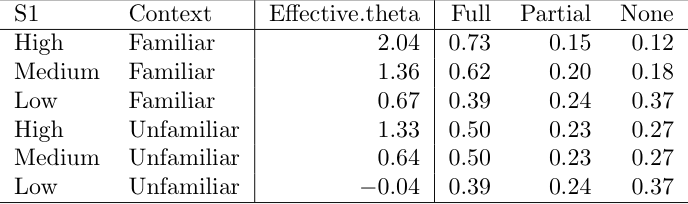# CPTtools framework

• Building a CPT requires three steps:
• Map each parent state into a effective theta for that parent
• Combine the parent effective thetas to an effective theta for each row of the CPT using one $$or more$$ combination rules
• Combination rules generally take one or more $$often one for each parent variable$$ discrimination parameters which weight the parent variable contributions $$log alphas$$
• Combination rules generally take one or more difficulty parameters $$often one for each state of the child variable$$ which shift the average probability of a correct response $$betas$$
• Map the effect theta for each row into a conditional probability of seeing each state using a link function
• Link functions can take a scaling parameter. $$link scale$$

# Parent level effective thetas

Effective theta scale is a logit scale corresponds to mean 0 SD 1 in a “standard” population.

Want the effective theta values to be equally spaced on this scale

Want the marginal distribution implied by the effective thetas to be uniform $$unit of the combination operator$$

What the effective theta transformation to be effectively invertible $$this is reason to add the 1\.7 to the IRT equation$$.

# Equally spaced quantiles of the normal distribution

Suppose variable has M states: 0,…,M-1

Want the midpoint of the interval going from probability m/M to $$m\+1$$/M .

Solution is to map state m onto

R code: qnorm$$\(1:M$$-.5)/M)